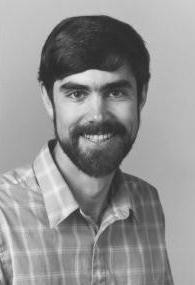# THEMATIC PROGRAMSOctober  4, 2023Numerical and Computational Challenges in Science and Engineering Program

### Randall J. LeVeque Applied Mathematics Department, University of Washington Solving Wave Propagation Problems in Heterogeneous Media

Abstract:
Wave propagation problems typically lead to hyperbolic systems of partial differential equations, with coefficients determined by the material properties of the medium through which the wave propagates. These coefficients determine the propagation speed and characteristic structure of the waves. Many practical problems require modeling the propagation of waves through heterogeneous media, in which the coefficients are spatially varying and often discontinuous across sharp interfaces between different materials. In these three lectures I will present an overview of some wave propagation problems and a class of numerical methods that can be used for their solution.

In the first lecture I will introduce the idea of a generalized Riemann problem at an interface between two materials and show how the wave structure of this Riemann solution relates to classical reflection and transmission coefficients. This viewpoint gives some insights into homogenization theory of waves in periodic media. This will be illustrated for an acoustics problem in one dimension. Nonlinear elastic wave propagation in a periodic medium will also be discussed, where the dispersive behavior caused by the periodic structure can lead to the appearance of solitary waves.

In the second lecture I will discuss high-resolution numerical methods based on the Riemann solution. These finite volume Godunov-type methods, originally developed for the accurate calculation of shock waves, are also useful for solving wave propagation problems in heterogeneous media. Nonlinear problems with spatially-varying flux functions can also be solved, including nonlinear elastic wave propagation problems.

The third lecture will concern multidimensional problems and numerical methods for their solution. The focus will be on acoustics and linear elastodynamic problems, though the methods can also be extended to multidimensional nonlinear problems.